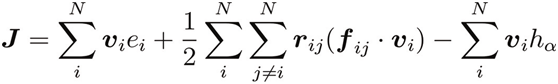# How to obtain the virial term

Hi, Eric Murphy
Thanks very much for you kindly help, as I described before:
For simulating the conductivity of nanofluid, I should subtract the last term from Heat Flux. As is liting in the formula below:The h in the last term describes in paper is sum of the average kinetic energy,potential energy and average virial terms per parcicle of each species.
I can’t get the virial term of per particle by lammps, could anyone give me some suggstion? thanks very much.
You may notice that the kinetic and potential are all scalar, I have noticed that the compute stress/atom gives a symmetric tensor, what I confused is how to sum them?
Or do I have something wrong with the formula?
Thanks for your time and information.
Best regards.

Hi, Eric Murphy
Thanks very much for you kindly help, as I described before:
For simulating the conductivity of nanofluid, I should subtract the
last term from Heat Flux. As is liting in the formula below:

​there is no formula.​

The h in the last term describes in paper is sum of the average kinetic
energy,potential energy and average virial terms per parcicle of
each species.
I can't get the virial term of per particle by lammps, could anyone give
me some suggstion? thanks very much.
You may notice that the kinetic and potential are all scalar, I have
noticed that the compute stress/atom gives a symmetric tensor, what I
confused is how to sum them?
Or do I have something wrong with the formula?

​why not simply use LAMMPS' native heat/flux compute​?
http://lammps.sandia.gov/doc/compute_heat_flux.html

axel.

Indeed. You might recognize the formula there. …+(stress Tensor)*vector=heat flux vector